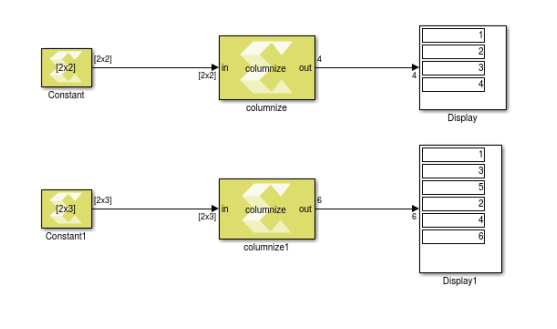# Defining Blocks Using Function Templates - 2022.1 English

## Vitis Model Composer User Guide (UG1483)

Document ID
UG1483
Release Date
2022-05-26
Version
2022.1 English
Important: To use template syntax, your function signature and definition should both be specified in a header file when running `xmcImportFunction`.

While it is common to write functions that accept only a predetermined data type, such as `int32`, in some cases you may want to create a block that accepts inputs of different sizes, or supports different data types, or create a block that accepts signals with different fixed-point lengths and fractional lengths. To do this you can use a function template that lets you create a block that accepts a variable signal size, data type, or data dimensions.

You can define blocks using function templates, as shown in the following example:

``````#include <stdint.h>
template <int ROWS, int COLS>
const int16_t in2[ROWS][COLS],
int16_t out[ROWS][COLS]) {
for (int i = 0; i<ROWS; i++) {
for (int j = 0; j<COLS; j++) {
out[i][j] = in1[i][j] + in2[i][j];
}
}
}``````

The example uses the template parameters `ROWS` and `COLS`. The actual dimensions of the input and output arrays, `in1[ROWS][COLS]` for instance, are determined at simulation time by the dimensions of the input signals to the block. `ROWS` and `COLS` are template parameters used to define the dimensions of the function arguments, and also used in the body of the function, `i<ROWS` for example.

Use the command below to import the function into Model Composer:
``````xmcImportFunction('SimpleLib',{'simple_matrix_add'},...
'template_example.h',{},{},'unlock')``````
Tip: In the example above the ellipsis (...) is used to indicate a continuation of the command on the next line. Refer to Continue Long Statements on Multiple Lines in the MATLAB documentation for more information.

You can perform simple arithmetic operations using template parameters. For example, the following code multiplies the ROWS and COLS of the input matrix to define the output, as shown in the figure below.

``````#include <stdint.h>
#pragma XMC INPORT in
#pragma XMC OUTPORT out
template<int ROWS,int COLS>
void columnize(const int16_t in[ROWS][COLS], int16_t out[ROWS*COLS]) {
for (int i = 0; i<ROWS; i++) {
for (int j = 0; j<COLS; j++) {
out[i*COLS+j] = in[i][j];
}
}
}``````
Figure 1. Columnize FunctionOther simple supported operations include +, -, *, /, %, <<, and >>, using both the template parameters and integer constants. For example:

``````template<int M, int N>
void func(const int in[M][N], int out[M*2][M*N]);

template<int ROWS, int COLS>
void func(array[2 * (ROWS + 1) + COLS + 3]);``````

You can also define a function template that uses a fixed-point data type of variable word length and integer length using function templates, as shown in the following example:

``````#include <stdint.h>
#include <ap_fixed.h>
#pragma XMC OUTPORT out
template <int WordLen, int IntLen>
The example above uses the fixed point notations from Vitis HLS, which specifies the word length and the integer length. In Model Composer, as described in Working with Data Types, you specify the word length and the fractional length. This requires you to use some care in connecting fixed point data types in Model Composer to the imported `fixed_add` block. For example, in the function above if `WordLen` is 16 and `IntLen` is 11, in Model Composer fixed point data type the word length is 16, and the fractional length is 5. For more information on fixed-point notation in Vitis HLS, refer to the Vitis High-Level Synthesis User Guide (UG1399).
To import the `fixed_add` function and create a block in Model Composer, use the following command:
``````xmcImportFunction('SimpleLib',{'fixed_add'},fixed_example.h',{},...